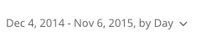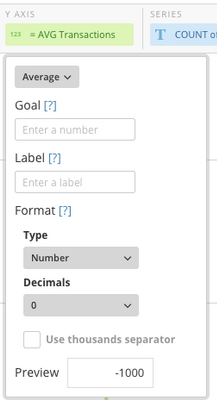# Calculating Xbar in Beast Mode

I am trying to create a control chart and need to start by calculating the Xbar.

In this particular dataset, I have multiple inventory transactions that have occurred during a "subgroup" = one day/week.  The Xbar calculation = the sum of the transactions during the subgroup period divided by the # transactions, by inventory class.

Nov 1.; Inventory Class1 transactions:  1200+1400+1000+800

Calculation :   4400/4 = 1100

Nov 2. ; Inventory Class1 transactions:  1000+700+1300

Calculation:  3000/3 = 1000

Nov 3.; Inventory Class1 transactions:   1200+500+500+1000

Calculation:  3200/4 = 800

The points I would want to plot on my chart would be 1100, 1000 and 800 for Inventory Class1.

What's the best way to go about accomplishing this?

Thanks!

JCB

• One of the great things about cards in Domo is their ability to dynamically group data based on a selected date grain. Your use case seems to be a good example of how to use that feature in a great way. If you set your date grain on the card to match your subgroup period ("by day" for example)and then choose "Average" as the aggregation option for the transactions field, it will automatically average the transactions according to the number of records that fall into each group.

Later, if you decide to change the date grain to weekly instead, the calculations will still work because the averaging will happen across the number of records in each week instead of each day.

If you want to do the averaging in a Beastmode, just wrap the field with the AVG function, e.g.

AVG(`Transactions`)

and the date grain will still apply to the beast mode.

Does that help?

If I have the data grouped by days but need the daily summed quantity averaged over the population, how do I do that?  Right now, when I bring in avg primary quantity, it is averaging the individual transactions - which I thought I wanted but it doesn't give me the trend line I was looking for.

• Just to clarify, are you saying you'd like to see the daily summation divided by the count of all records on the dataset?

If so, it would need to be done in a dataflow instead of beastmode, I believe. Beastmode calculations are done on a group by group basis. You can't reference an aggregation on the group (daily sum) and the total (Count of all records) at the same time.

In a dataflow, you could write a transform to get you the count of the records in the population, another to give you the aggregated data for each day using a Group By, then join the two together to get the fields in one place for the calculation. Alternatively, you could write a transform to get your population total and then join it to all records in your dataset. Then in your beast mode, you could use something like

SUM(`Transactions`) / MAX(`Population`)

with your date grain set to daily. Then you'd end up with your daily total divided by your population.

Does that get you any closer?

• Thanks for the reply -

I'd like to use the summation of the daily transactions to determine the average for a different timeframe i.e. weekly or monthly.

So, if I want to see the data by day for 30 days, I would want to plot the summation of the daily inventories and use those to calculate the average over the month.

Instead, I'm getting the average of the daily transactions, which are high volume but low quantities and are not giving me the trend line I'm looking for.  It makes sense what you're saying about Beast Mode calculations only being done on a group by group basis - this explains why I can get the daily summation but not the weekly average.

I'll explore the dataflow and see what I can do there.  Thanks for again for the recommendation!

J.

This discussion has been closed.